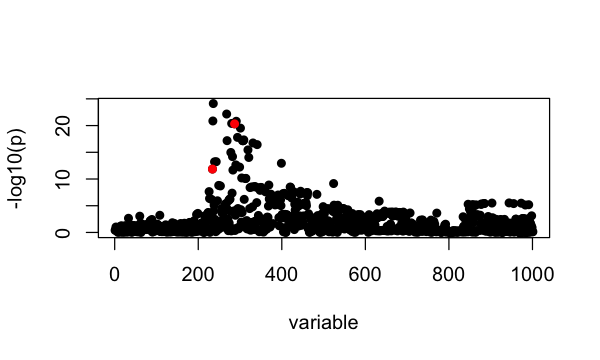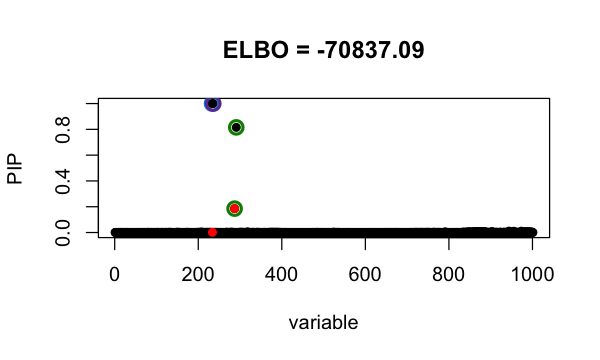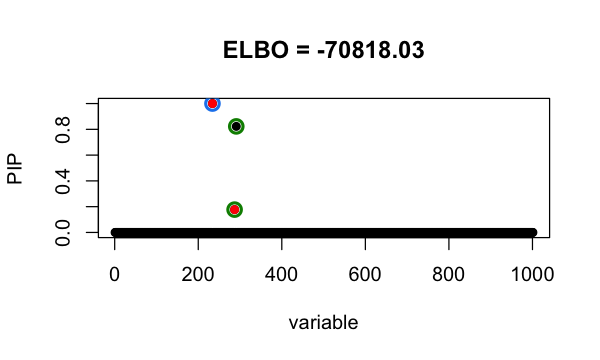# Refine SuSiE model

#### 2021-11-12

In this vignette, we demonstrate a procedure that helps SuSiE get out of local optimum.

We simulate phenotype using UK Biobank genotypes from 50,000 individuals. There are 1001 SNPs. It is simulated to have exactly 2 non-zero effects at 234, 287.

library(susieR)
library(curl)
data_file <- tempfile(fileext = ".RData")
data_url <- paste0("https://raw.githubusercontent.com/stephenslab/susieR/",
"master/inst/datafiles/FinemappingConvergence1k.RData")
b <- FinemappingConvergence$true_coef susie_plot(FinemappingConvergence$z, y = "z", b=b)The strongest marginal association is a non-effect SNP.

Since the sample size is large, we use sufficient statistics ($$X^\intercal X, X^\intercal y, y^\intercal y$$ and sample size $$n$$) to fit susie model. It identifies 2 Credible Sets, one of them is false positive. This is because susieR get stuck around a local minimum.

fitted <- with(FinemappingConvergence,
susie_suff_stat(XtX = XtX, Xty = Xty, yty = yty, n = n))
susie_plot(fitted, y="PIP", b=b, main=paste0("ELBO = ", round(susie_get_objective(fitted),2)))Our refine procedure to get out of local optimum is

1. fit a susie model, $$s$$ (suppose it has $$K$$ CSs).

2. for CS in $$s$$, set SNPs in CS to have prior weight 0, fit susie model –> we have K susie models: $$t_1, \cdots, t_K$$.

3. for each $$k = 1, \cdots, K$$, fit susie with initialization at $$t_k$$ ($$\alpha, \mu, \mu^2$$) –> $$s_k$$

4. if $$\max_k \text{elbo}(s_k) > \text{elbo}(s)$$, set $$s = s_{kmax}$$ where $$kmax = \arg_k \max \text{elbo}(s_k)$$ and go to step 2; if no, break.

We fit susie model with above procedure by setting refine = TRUE.

fitted_refine <- with(FinemappingConvergence,
susie_suff_stat(XtX = XtX, Xty = Xty, yty = yty,
n = n, refine=TRUE))
# XtX is not symmetric; forcing XtX to be symmetric by  replacing XtX with (XtX + t(XtX))/2
# XtX is not symmetric; forcing XtX to be symmetric by  replacing XtX with (XtX + t(XtX))/2
# XtX is not symmetric; forcing XtX to be symmetric by  replacing XtX with (XtX + t(XtX))/2
# XtX is not symmetric; forcing XtX to be symmetric by  replacing XtX with (XtX + t(XtX))/2
# XtX is not symmetric; forcing XtX to be symmetric by  replacing XtX with (XtX + t(XtX))/2
# XtX is not symmetric; forcing XtX to be symmetric by  replacing XtX with (XtX + t(XtX))/2
# XtX is not symmetric; forcing XtX to be symmetric by  replacing XtX with (XtX + t(XtX))/2
# XtX is not symmetric; forcing XtX to be symmetric by  replacing XtX with (XtX + t(XtX))/2
# XtX is not symmetric; forcing XtX to be symmetric by  replacing XtX with (XtX + t(XtX))/2
# XtX is not symmetric; forcing XtX to be symmetric by  replacing XtX with (XtX + t(XtX))/2
susie_plot(fitted_refine, y="PIP", b=b, main=paste0("ELBO = ", round(susie_get_objective(fitted_refine),2)))With the refine procedure, it identifies 2 CSs with the true signals, and the achieved evidence lower bound (ELBO) is higher.

## Session information

Here are some details about the computing environment, including the versions of R, and the R packages, used to generate these results.

sessionInfo()
# R version 3.6.2 (2019-12-12)
# Platform: x86_64-apple-darwin15.6.0 (64-bit)
# Running under: macOS Catalina 10.15.7
#
# Matrix products: default
# BLAS:   /Library/Frameworks/R.framework/Versions/3.6/Resources/lib/libRblas.0.dylib
# LAPACK: /Library/Frameworks/R.framework/Versions/3.6/Resources/lib/libRlapack.dylib
#
# locale:
#  en_US.UTF-8/en_US.UTF-8/en_US.UTF-8/C/en_US.UTF-8/en_US.UTF-8
#
# attached base packages:
#  stats     graphics  grDevices utils     datasets  methods   base
#
# other attached packages:
#  curl_4.3       Matrix_1.2-18  L0Learn_1.2.0  susieR_0.11.92
#
# loaded via a namespace (and not attached):
#   Rcpp_1.0.7         highr_0.8          plyr_1.8.5         compiler_3.6.2
#   pillar_1.6.2       tools_3.6.2        digest_0.6.23      evaluate_0.14
#   lifecycle_1.0.0    tibble_3.1.3       gtable_0.3.0       lattice_0.20-38
#  pkgconfig_2.0.3    rlang_0.4.11       DBI_1.1.0          parallel_3.6.2
#  yaml_2.2.0         xfun_0.11          stringr_1.4.0      dplyr_1.0.7
#  knitr_1.26         generics_0.0.2     vctrs_0.3.8        RcppZiggurat_0.1.5
#  Rfast_2.0.3        grid_3.6.2         tidyselect_1.1.1   reshape_0.8.8
#  glue_1.4.2         R6_2.4.1           fansi_0.4.0        rmarkdown_2.3
#  mixsqp_0.3-46      irlba_2.3.3        reshape2_1.4.3     ggplot2_3.3.5
#  purrr_0.3.4        magrittr_2.0.1     matrixStats_0.61.0 scales_1.1.0
#  htmltools_0.4.0    ellipsis_0.3.2     assertthat_0.2.1   colorspace_1.4-1
#  utf8_1.1.4         stringi_1.4.3      munsell_0.5.0      crayon_1.4.1